# DAV Class 6 Maths Chapter 2 Worksheet 6 Solutions

The DAV Class 6 Maths Book Solutions Pdf and DAV Class 6 Maths Chapter 2 Worksheet 6 Solutions of Factors and Multiples offer comprehensive answers to textbook questions.

## DAV Class 6 Maths Ch 2 WS 6 Solutions

Question 1.
Find LCM of the following numbers by taking their multiples.

(a) 12, 9
Solution:
12, 9
Multiples of 12 are 12, 24, 36, 48 …..
Multiples of 9 are 9, 18, 27, 36, 45 …
Least common multiple of 12 and 9 is 36
Hence, the required LCM = 36.

(b) 4, 5, 2
Solution:
4, 5, 2
Multiples of 4 are 4, 8, 12, 16, 20, 24 …..
Multiples of 5 are 5, 10, 15, 20, 25, 30 …..
Multiples of 2 are 2, 4, 6, 8, 10, 12, 14, 16, 18, 20, 22 …..
Least common multiples of 4, 5 and 2 = 20
Hence, the required LCM = 20.

(c) 25, 15
Solution:
25, 15
Multiples of 25 are 25, 50, 75, 100 …..
Multiples of 15 are 15, 30, 45, 75, 90, 105 …..
Least common multiples of 25 and 15 is 75
Hence, the required LCM = 75.Question 2.
Find the LCM by prime factorisation method.
(a) 10, 15, 6
Solution:
Prime factors of 10, 15 and 6 are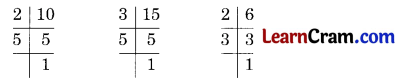10 = 2 × 5
15 = 3 × 5
6 = 2 × 3
LCM = 2 × 3 × 5 = 30
Hence the required LCM = 30.

(b) 16, 12, 18
Solution:
Prime factors of 16, 12, 18 are16 = 2 × 2 × 2 × 2
12 = 2 × 2 × 3
18 = 2 × 3 × 3
LCM = 2 × 2 × 2 × 2 × 3 × 3 = 144
Hence the required LCM = 144.

(c) 25, 30, 40
Solution:
Prime factors of 25, 30, 40 are25 = 5 × 5
30 = 2 × 3 × 5
40 = 2 × 2 × 2 × 5
LCM = 2 × 2 × 2 × 3 × 5 × 5 = 600
Hence, the required LCM = 600.Question 3.
Find the LCM by common division method.
(a) 12, 15, 45LCM = 2 × 2 × 3 × 3× 5 = 180
Hence, the required LCM = 180

(b) 24, 90, 48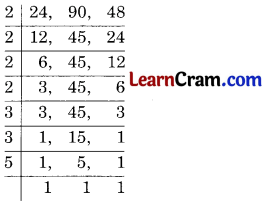LCM = 2 × 2 × 2 × 2 × 3 × 3 × 5 = 720
Hence, the required LCM = 720

(c) 30, 24, 36, 16
Solution:LCM = 2 × 2 × 2 × 2 × 3 × 3 × 5 = 720
Hence, the required LCM = 720(d) 16, 48, 64
Solution:LCM = 2 × 2 × 2 × 2 × 2 × 2 × 3 = 192
Hence, the required LCM = 192

(e) 35, 49, 91
Solution: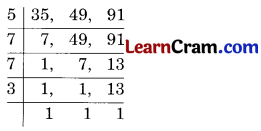LCM = 5 × 7 × 7 × 13 = 3185
Hence, the required LCM = 3185

(f) 40, 48, 445
Solution: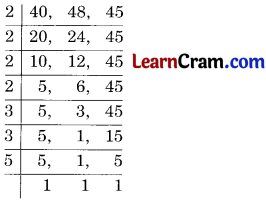LCM = 2 × 2 × 3 × 5 × 5 = 300
Hence, the required LCM = 300(g) 12, 16, 24, 36
Solution:LCM = 2 × 2 × 2 × 2 × 3 × 3 = 144
Hence, the required LCM = 144

(h) 40, 48, 45
Solution: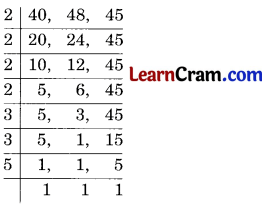LCM = 2 × 2 × 2 × 2 × 3 × 3 × 5 = 720
Hence, the required LCM = 720Question 4.
Find the least number which when divided by 40, 50 and 60 leaves remainder 5 in each case.
Solution:
L.C.M. of 40, 50 and 60 isL.C.M. = 2 × 2 × 2 × 3 × 5 × 5 = 600
Remainder is 5
Hence, the required number = 600 + 5 = 605

Question 5.
Three Haryana Roadways buses stop after 50, 100 and 125 km respectively. If they leave together, then after how many kilometers will they stop together?
Solution:
LCM of 50, 100 and 125 isL.C.M. = 2 × 2 × 5 × 5 × 5 = 500
Hence, the required kilometers = 500 kmQuestion 6.
Four bells toll at intervals of 8, 9, 12 and 15 minutes respectively. If they toll together at 3 p.m., when will they toll together next?
Solution:
LCM of 8, 9, 12, 15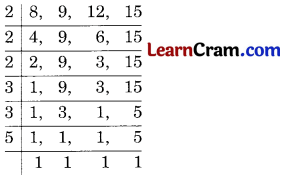LCM = 2 × 2 × 2 × 3 × 3 × 5 = 360 minutes
= $$\frac{360}{60}$$ = 6 hours
Hence, the required time = 3 + 6 = 9 p.m.